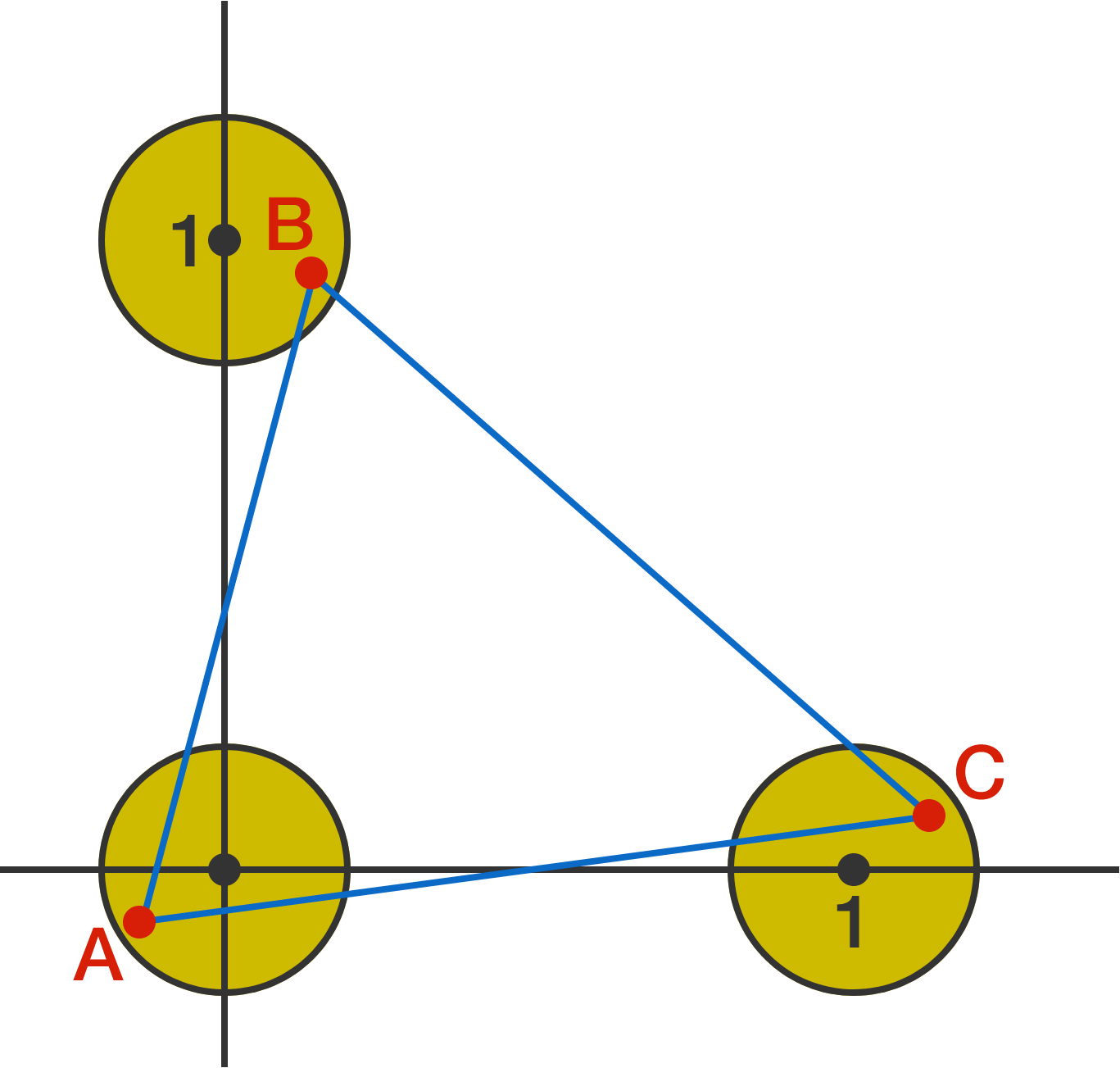# Three circles and a triangle

Geometry Level 4

Three circles with equal radii are centered at $(1,0), (0,0),$ and $(0,1)$.To 4 decimal places, what is the smallest radius of the circles such that we can pick a point within each circle--like A, B, C in the diagram--and connect them to form an equilateral triangle?

×